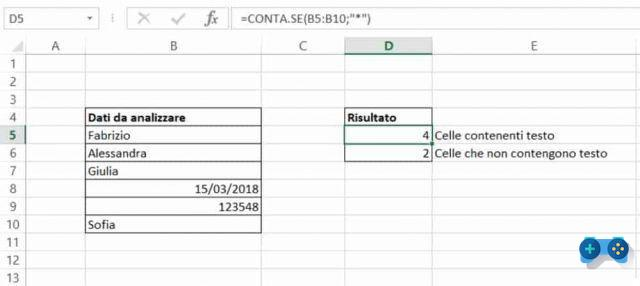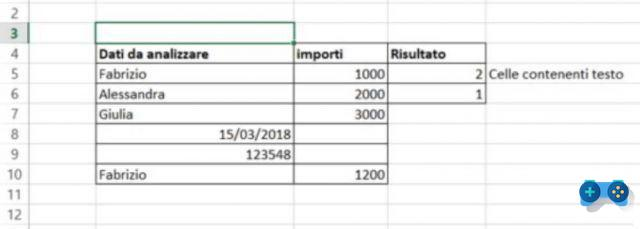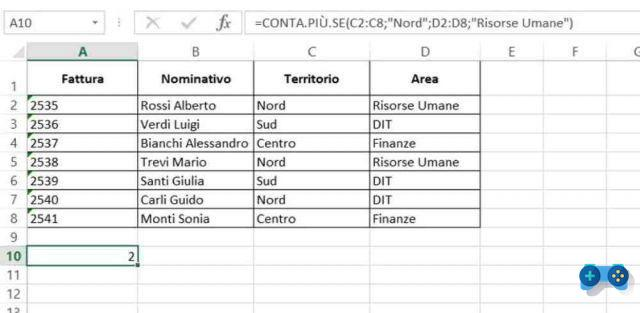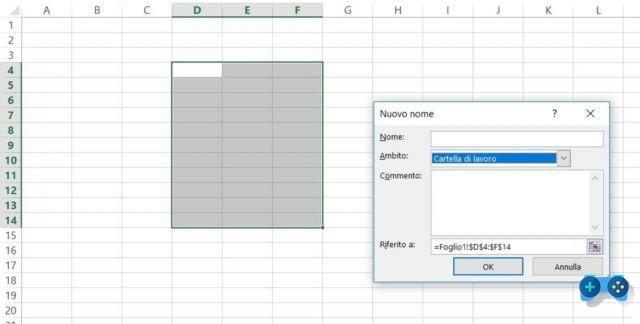# How to count cells with text in ExcelWho I am
Lluis Enric Mayans
@lluisenricmayansAuthor and references

How to count cells with text in Excel. Here is a simple guide that reveals a trick to count the number of cells containing text through one of the many Excel functions.

Are you trying to figure out in your Excel sheet how many times a text value occurs in a specific cell range? If you don't know there are several ways to count cells with text in Excel. Therefore? Get comfortable and relax and start Excel, because by following this guide you will be able in no time to use those functions that will allow you to easily apply what you are trying to do.

Are you ready? Let's see what are the functions that will allow you to count if the cell contains text or part of a text.## CONTA.SE

The function CONTA.SE can be used to determine how many times the text is present and how many times the text is not present. Here is an example of what counts if:The syntax to apply to determine how many cells contain text with count if Excel, in this example, is as follows: =ACCOUNT IF(B5:B10;"*"). In this case the asterisk is a wildcard that matches any number of text characters.

If, on the other hand, you want to determine how many cells do not contain text, the syntax to apply is the following: =ACCOUNT IF(B5:B10;"<>*").

In case you need to determine how many times a text repeats in a specific range of cells, just replace the wildcard with the text to search for. Eg: = COUNTIF (B5: B10; "Fabrizio"), will search for the text Fabrizio in the specified cell range and give a count as a result.Other customizations are:

• =ACCOUNT IF(B5:B10;B6), to determine the number of voices in B6.
• =ACCOUNT IF(C5:C10;"<2000″), to determine the number of amounts below 2000.
• =ACCOUNT IF(C5:C10;">="&C6), to determine the number of amounts above 2000.

## CONTA.PIU.SE

The function COUNTIFS it is similar to the function CONTA.SE, with the only difference that it can be applied to multiple cell ranges by counting the number of times the criteria are met.COUNTIFS check for "North part"In the first interval and"Resources humanity”In the second interval, and displays 2, the total number of employees in the Human Resources Area for the Northern Territory.How to name cells in Excel

## IF AND SUM

IF and SUM function is another solution to count cells with text in Excel. The proposed solution is for Excel experts and requires careful knowledge of the Microsoft tool.

To get the desired result, use the function first SE, to test the values ​​in the cells, and if the test result is True, with the function SUM the sum of the values ​​that passed the test will be made.

So in our case, to calculate the number of occurrences of the text "North part"And the text"South“, Of the column Territory, you will have to do =SOMMA(SE((C2:C8=”Nord”)+(C2:C8=”Sud”);1;0)).

Attention to get the result 5 (3 North + 2 South) you will need to enter this formula as an array formula. On Windows 10 you have to press F2 and then simultaneously CTRL + SHIFT + ENTER to make the formula return the desired results. On MAC you have to use CMD + SHIFT + ENTER.

Excel will automatically put curly brackets on the formula to indicate that it is an array formula.How to compare two tables in Excel

### Related Items

##### add a comment of How to count cells with text in Excel
Comment sent successfully! We will review it in the next few hours.